CBSE Class 12 Sample Paper for 2023 Boards

Class 12
Solutions of Sample Papers and Past Year Papers - for Class 12 Boards

## An insect is crawling along the line insect is crawling along the line r = 6i ̂+2j ̂+2k ̂+λ(i ̂-2j ̂+2k ̂ ) and another insect is crawling along the line r = −4i ̂-k ̂+μ(3i ̂-2j ̂-2k ̂ ). At what points on the lines should they reach so that the distance between them is the shortest? possible distance between them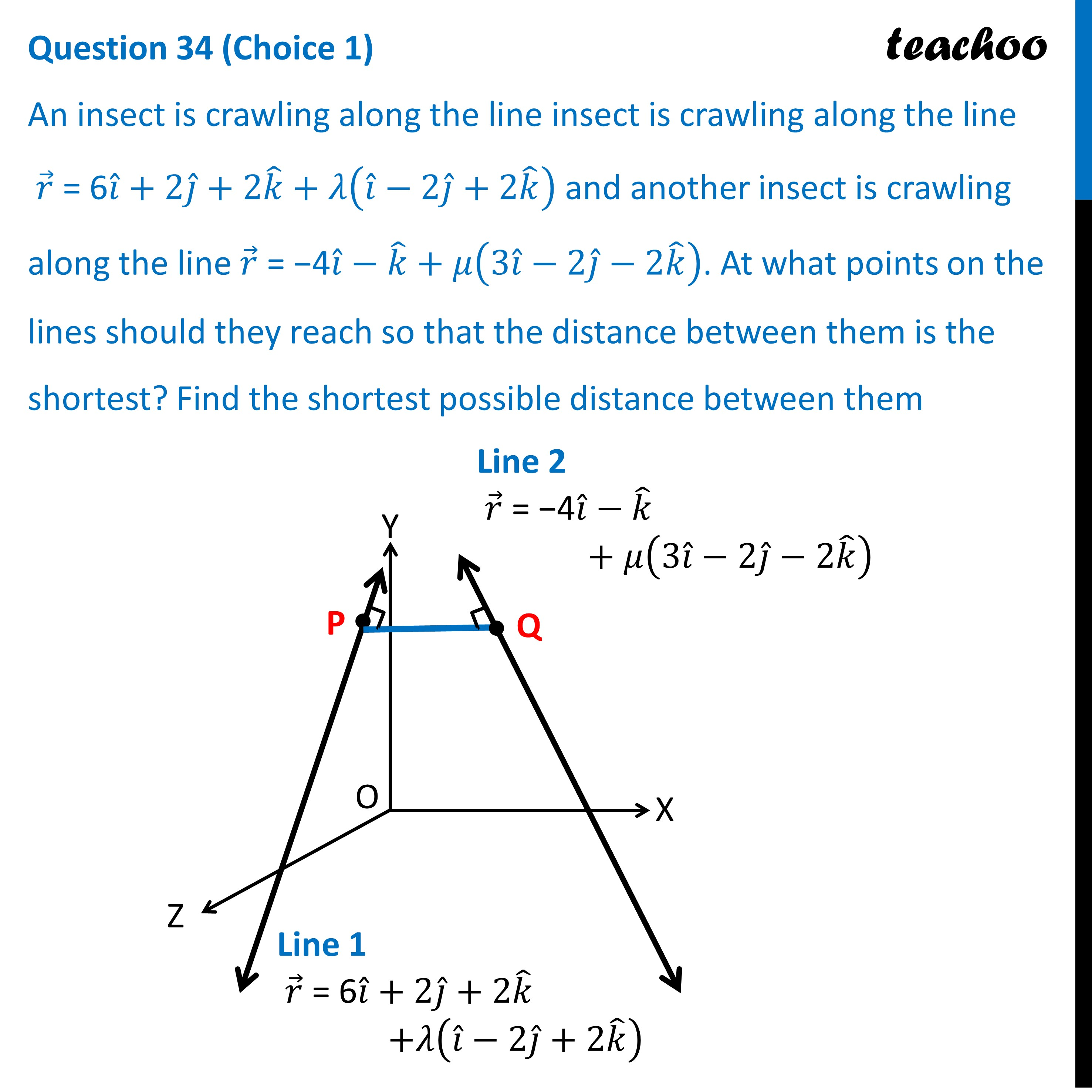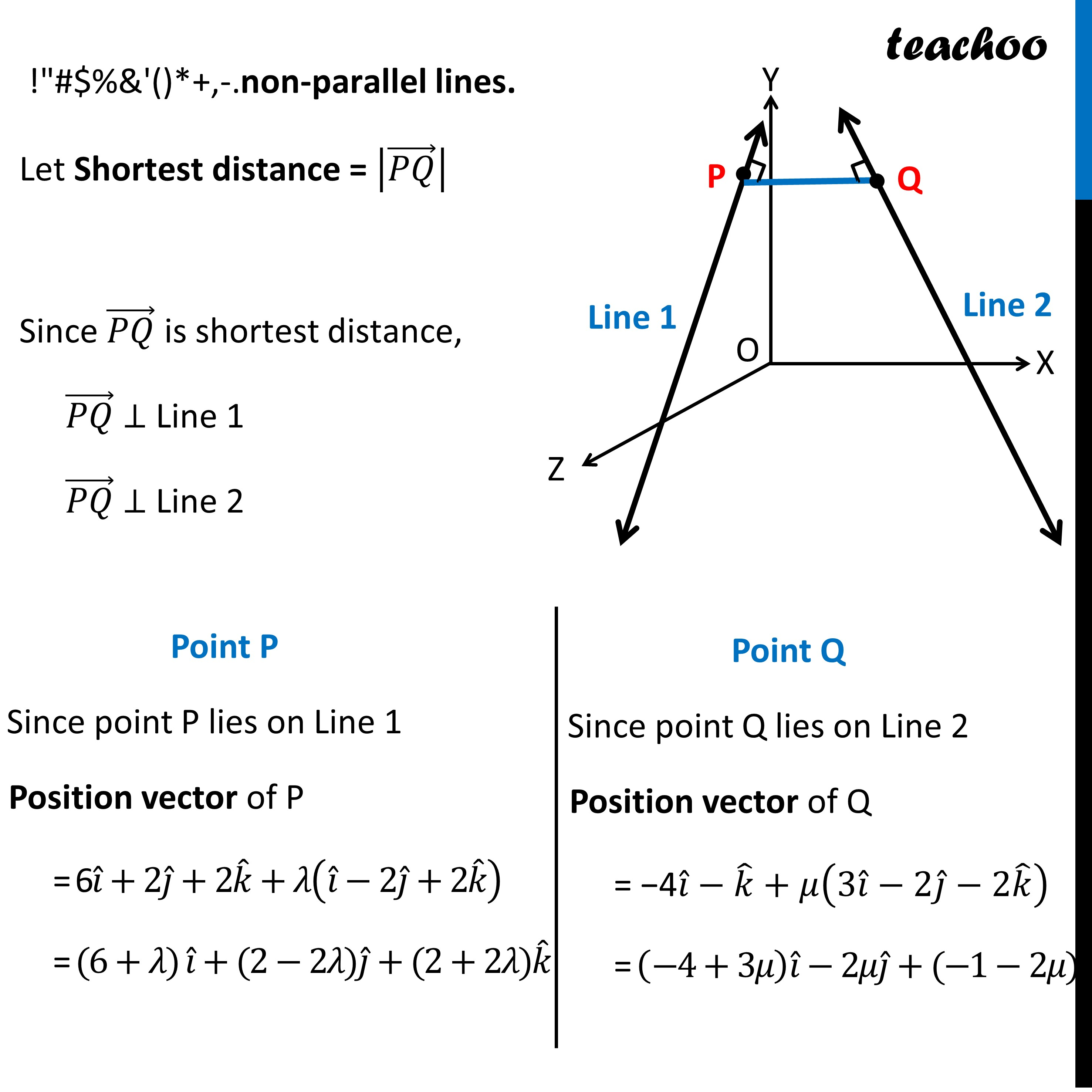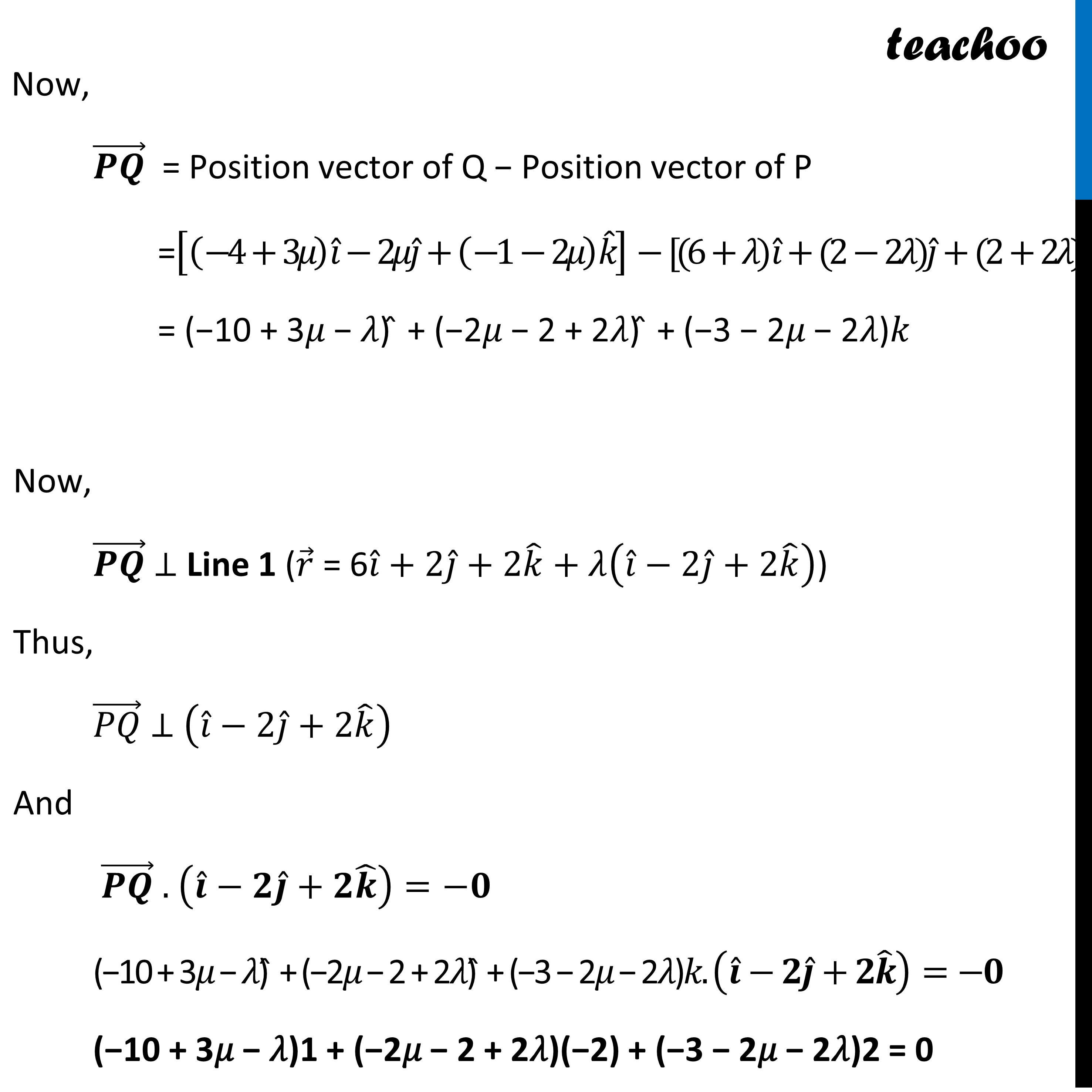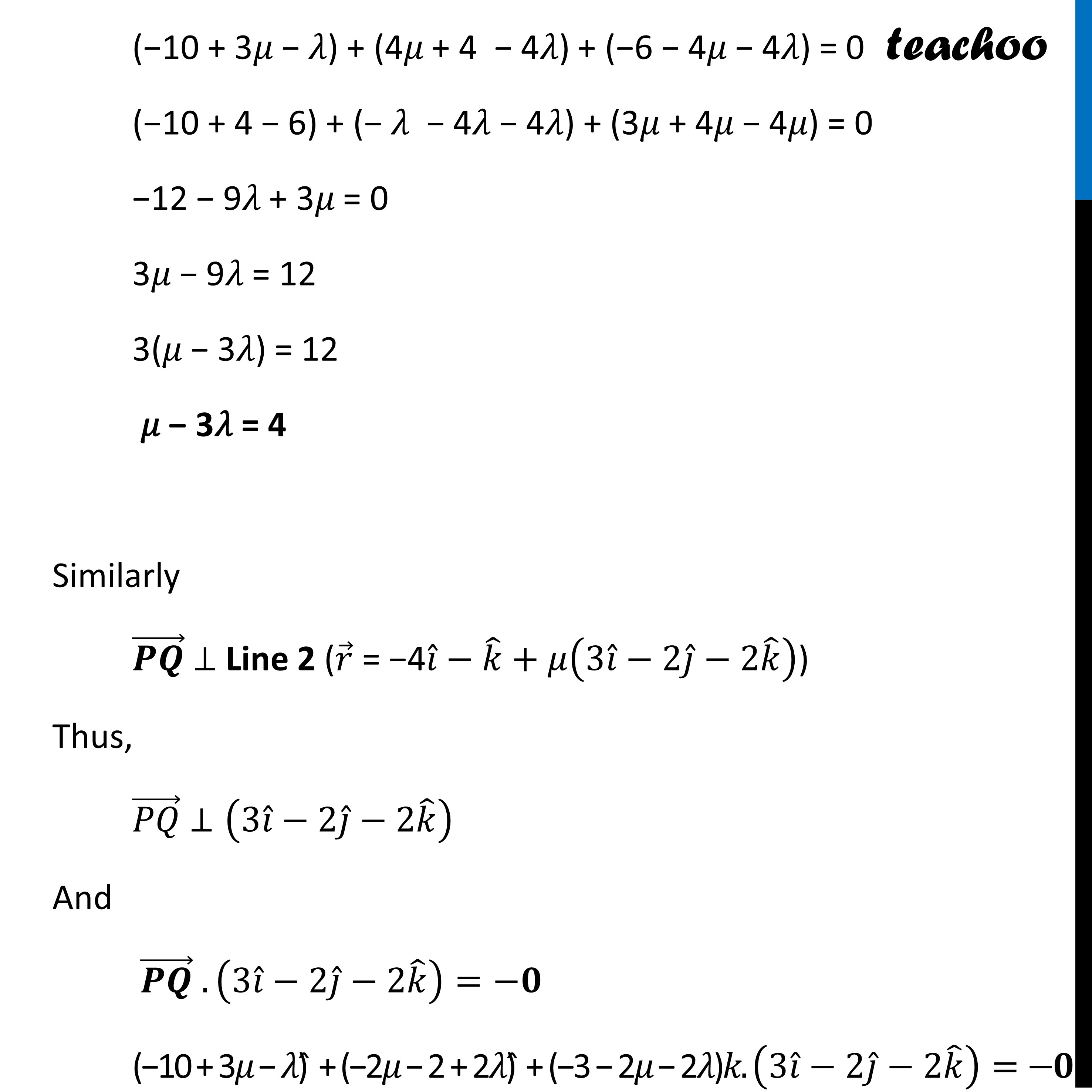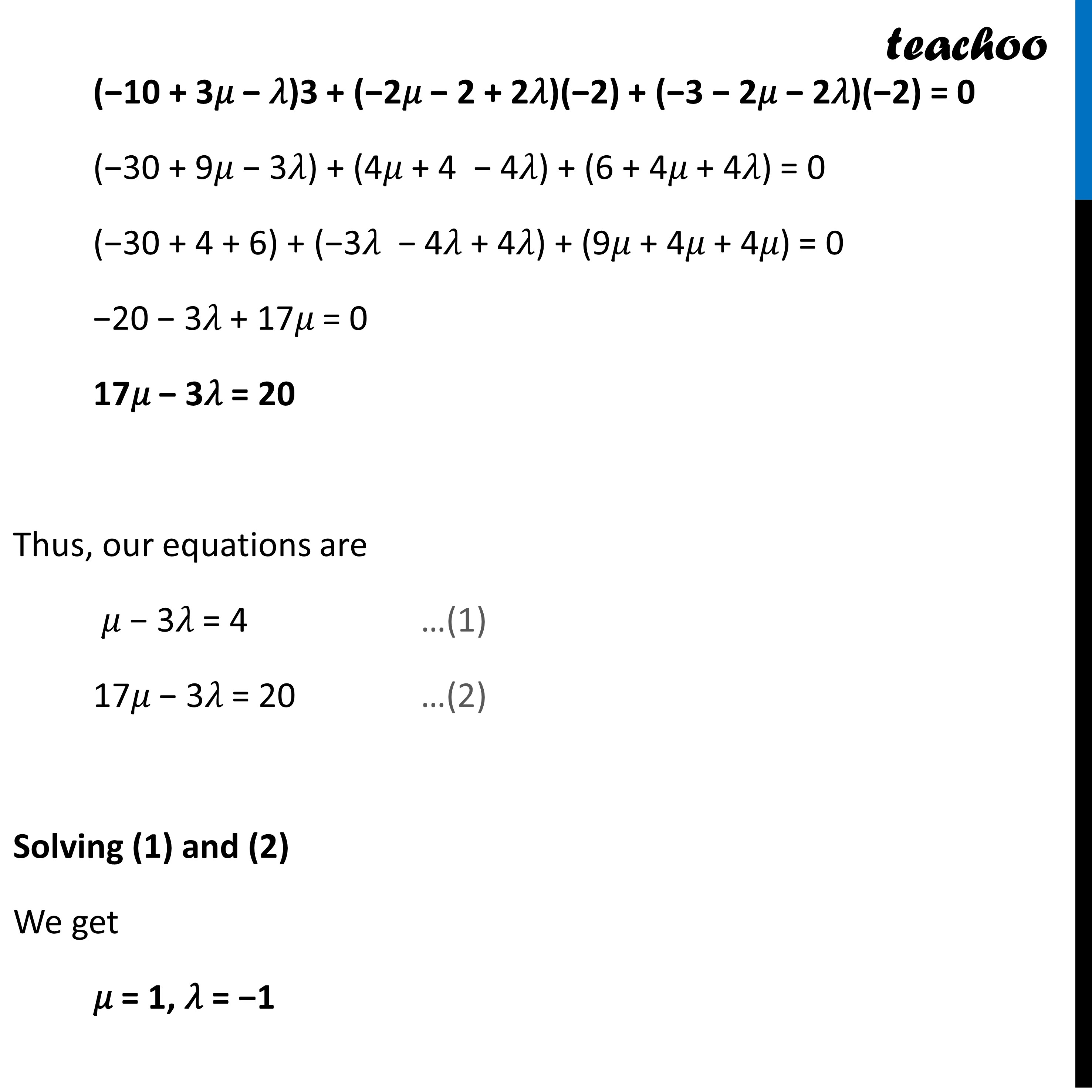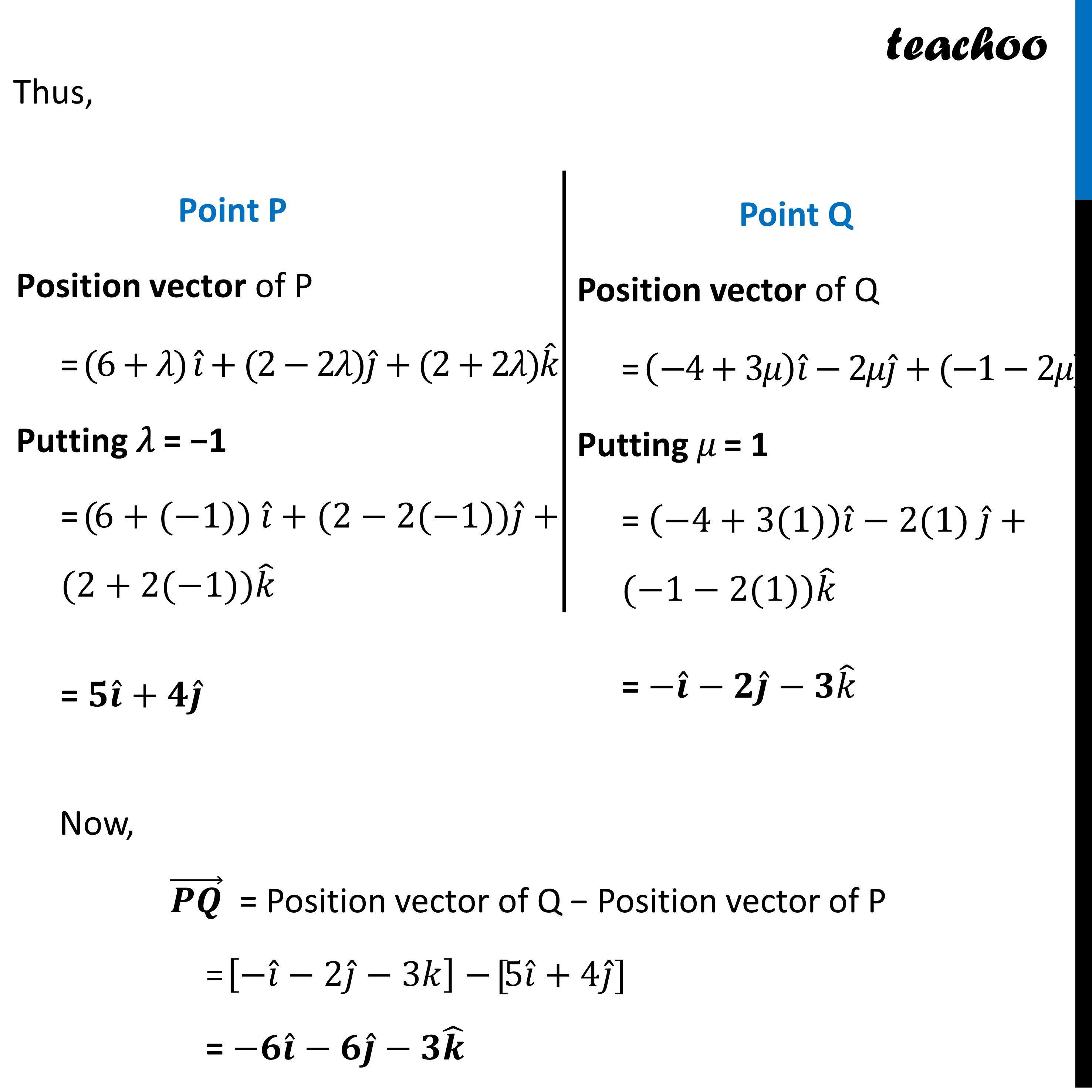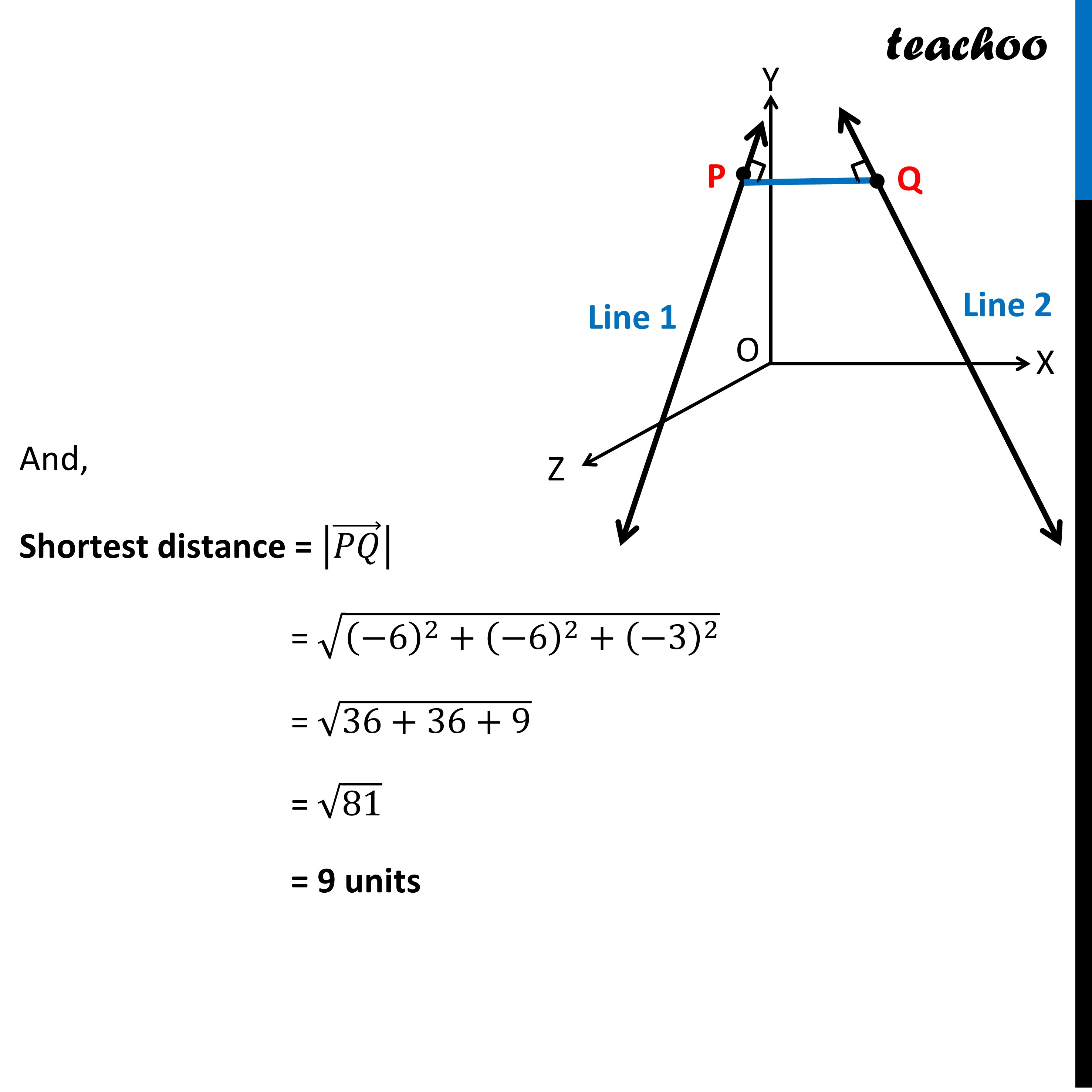Learn in your speed, with individual attention - Teachoo Maths 1-on-1 Class

### Transcript

Question 34 (Choice 1) An insect is crawling along the line insect is crawling along the line 𝑟 ⃗ = 6𝑖 ̂+2𝑗 ̂+2𝑘 ̂+𝜆(𝑖 ̂−2𝑗 ̂+2𝑘 ̂ ) and another insect is crawling along the line 𝑟 ⃗ = −4𝑖 ̂−𝑘 ̂+𝜇(3𝑖 ̂−2𝑗 ̂−2𝑘 ̂ ). At what points on the lines should they reach so that the distance between them is the shortest? Find the shortest possible distance between them Line 2 𝑟 ⃗ = −4𝑖 ̂−𝑘 ̂ + 𝜇(3𝑖 ̂−2𝑗 ̂−2𝑘 ̂ ) Line 1 𝑟 ⃗ = 6𝑖 ̂+2𝑗 ̂+2𝑘 ̂ +𝜆(𝑖 ̂−2𝑗 ̂+2𝑘 ̂ ) ﻿The given lines are non-parallel lines. Let Shortest distance = |(𝑃𝑄) ⃗ | Since (𝑃𝑄) ⃗ is shortest distance, (𝑃𝑄) ⃗ ⊥ Line 1 (𝑃𝑄) ⃗ ⊥ Line 2 Point P Since point P lies on Line 1 Position vector of P = 6𝑖 ̂+2𝑗 ̂+2𝑘 ̂+𝜆(𝑖 ̂−2𝑗 ̂+2𝑘 ̂ ) = (6+𝜆) 𝑖 ̂+(2−2𝜆)𝑗 ̂+(2+2𝜆)𝑘 ̂ Point Q Since point Q lies on Line 2 Position vector of Q = −4𝑖 ̂−𝑘 ̂+𝜇(3𝑖 ̂−2𝑗 ̂−2𝑘 ̂ ) = (−4+3𝜇) 𝑖 ̂−2𝜇𝑗 ̂+(−1−2𝜇)𝑘 ̂ Now, (𝑷𝑸) ⃗ = Position vector of Q − Position vector of P = [(−4+3𝜇) 𝑖 ̂−2𝜇𝑗 ̂+(−1−2𝜇) 𝑘 ̂ ]−[(6+𝜆) 𝑖 ̂+(2−2𝜆)𝑗 ̂+(2+2𝜆)𝑘 ̂] = ﻿(−10 + 3𝜇 − 𝜆)𝚤̂ + (−2𝜇 − 2 + 2𝜆)𝚥̂ + (−3 − 2𝜇 − 2𝜆)𝑘 Now, (𝑷𝑸) ⃗ ⊥ Line 1 (𝑟 ⃗ = 6𝑖 ̂+2𝑗 ̂+2𝑘 ̂+𝜆(𝑖 ̂−2𝑗 ̂+2𝑘 ̂ )) Thus, (𝑃𝑄) ⃗ ⊥ (𝑖 ̂−2𝑗 ̂+2𝑘 ̂ ) And (𝑷𝑸) ⃗ . (𝒊 ̂−𝟐𝒋 ̂+𝟐𝒌 ̂ )=−𝟎 (−10 + 3𝜇 − 𝜆)𝚤̂ + (−2𝜇 − 2 + 2𝜆)𝚥̂ + (−3 − 2𝜇 − 2𝜆)𝑘. (𝒊 ̂−𝟐𝒋 ̂+𝟐𝒌 ̂ )=−𝟎 (−10 + 3𝜇 − 𝜆)1 + (−2𝜇 − 2 + 2𝜆)(−2) + (−3 − 2𝜇 − 2𝜆)2 = 0 (−10 + 3𝜇 − 𝜆) + (4𝜇 + 4 − 4𝜆) + (−6 − 4𝜇 − 4𝜆) = 0 (−10 + 4 − 6) + (− 𝜆 − 4𝜆 − 4𝜆) + (3𝜇 + 4𝜇 − 4𝜇) = 0 −12 − 9𝜆 + 3𝜇 = 0 3𝜇 − 9𝜆 = 12 3(𝜇 − 3𝜆) = 12 𝜇 − 3𝜆 = 4 Similarly (𝑷𝑸) ⃗ ⊥ Line 2 (𝑟 ⃗ = −4𝑖 ̂−𝑘 ̂+𝜇(3𝑖 ̂−2𝑗 ̂−2𝑘 ̂ )) Thus, (𝑃𝑄) ⃗ ⊥(3𝑖 ̂−2𝑗 ̂−2𝑘 ̂ ) And (𝑷𝑸) ⃗ .(3𝑖 ̂−2𝑗 ̂−2𝑘 ̂ )=−𝟎 (−10 + 3𝜇 − 𝜆)𝚤̂ + (−2𝜇 − 2 + 2𝜆)𝚥̂ + (−3 − 2𝜇 − 2𝜆)𝑘.(3𝑖 ̂−2𝑗 ̂−2𝑘 ̂ )=−𝟎 (−10 + 3𝜇 − 𝜆)3 + (−2𝜇 − 2 + 2𝜆)(−2) + (−3 − 2𝜇 − 2𝜆)(−2) = 0 (−30 + 9𝜇 − 3𝜆) + (4𝜇 + 4 − 4𝜆) + (6 + 4𝜇 + 4𝜆) = 0 (−30 + 4 + 6) + (−3𝜆 − 4𝜆 + 4𝜆) + (9𝜇 + 4𝜇 + 4𝜇) = 0 −20 − 3𝜆 + 17𝜇 = 0 17𝜇 − 3𝜆 = 20 Thus, our equations are 𝜇 − 3𝜆 = 4 …(1) 17𝜇 − 3𝜆 = 20 …(2) Solving (1) and (2) We get 𝜇 = 1, 𝜆 = −1 Point P Position vector of P = (6+𝜆) 𝑖 ̂+(2−2𝜆)𝑗 ̂+(2+2𝜆)𝑘 ̂ Putting 𝜆 = −1 = (6+(−1)) 𝑖 ̂+(2−2(−1))𝑗 ̂+(2+2(−1))𝑘 ̂ = 𝟓𝒊 ̂+𝟒𝒋 ̂ Point Q Position vector of Q = (−4+3𝜇) 𝑖 ̂−2𝜇𝑗 ̂+(−1−2𝜇)𝑘 ̂ Putting 𝜇 = 1 = (−4+3(1)) 𝑖 ̂−2(1) 𝑗 ̂+(−1−2(1))𝑘 ̂ = −𝒊 ̂−𝟐𝒋 ̂−𝟑𝑘 ̂Now, (𝑷𝑸) ⃗ = Position vector of Q − Position vector of P = [−𝑖 ̂−2𝑗 ̂−3𝑘]−[5𝑖 ̂+4𝑗 ̂] = −𝟔𝒊 ̂−𝟔𝒋 ̂−𝟑𝒌 ̂ And, Shortest distance = |(𝑃𝑄) ⃗ | = √((−6)^2+(−6)^2+(−3)^2 ) = √(36+36+9) = √81 = 9 units